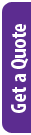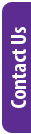## Details

Machine Learning is a branch of computer science that uses algorithms to help artificial intelligent systems learn and adapt. That learning process requires data and a programming language to process it. R is a language built for data science which has many packages to for machine learning, ready for you to use.

In this course you will learn:

• Key concepts and terms for machine learning
• To remove noise in data via smoothing
• To assess the effectiveness of your model with cross-validation
• To integrate model development using the Caret Package
• Useful algorithms for important tasks
• To apply machine learning to real-world applications
• How to work with large data sets

### Delivery Methods

Leading Training is focusing on providing virtual training courses for the foreseeable future and will only consider in-person and classroom training on request, with a required minimum group size of four delegates. We remain committed to offering training that is fast, focused and effective.

Delivery Method Duration Price (excl. VAT)
Fulltime Days R 28,990.00
Webinar Days R 23,500.00

### Brochure:

Information may change without notice.

## Audience

• Data scientists
• Programmers
• Engineers
• Scientists

## Pre-Requisites

Leading Training's Introduction to R Programming or equivalent knowledge

## Course Outline / Curriculum

Introduction to machine learning

• Notation
• An example
• Exercises
• Evaluation metrics
• Training and test sets
• Overall accuracy
• The confusion matrix
• Sensitivity and specificity
• Balanced accuracy and F1 score
• Prevalence matters in practice
• ROC and precision-recall curves
• The loss function
• Exercises
• Conditional probabilities and expectations
• Conditional probabilities
• Conditional expectations
• Conditional expectation minimizes squared loss function
• Exercises
• Case study: is it a 2 or a 7?

Smoothing

• Bin smoothing
• Kernels
• Local weighted regression (loess)
• Fitting parabolas
• Beware of default smoothing parameters
• Connecting smoothing to machine learning
• Exercises

Cross validation

• Motivation with k-nearest neighbors
• Over-training
• Over-smoothing
• Picking the k in kNN
• Mathematical description of cross validation
• K-fold cross validation
• Exercises
• Bootstrap
• Exercises

The caret package

• The caret train functon
• Cross validation
• Example: fitting with loess

Examples of algorithms

• Linear regression
• The predict function
• Exercises
• Logistic regression
• Generalized linear models
• Logistic regression with more than one predictor
• Exercises
• k-nearest neighbors
• Exercises
• Generative models
• Naive Bayes
• Controlling prevalence
• Linear discriminant analysis
• Connection to distance
• Case study: more than three classes
• Exercises
• Classification and regression trees (CART)
• The curse of dimensionality
• CART motivation
• Regression trees
• Classification (decision) trees
• Random forests
• Exercises
• Machine learning in practice
• Preprocessing
• k-nearest neighbor and random forest
• Variable importance
• Visual assessments
• Ensembles
• Exercises
• Large datasets
• Matrix algebra
• Notation
• Converting a vector to a matrix
• Row and column summaries
• apply
• Filtering columns based on summaries
• Indexing with matrices
• Binarizing the data
• Vectorization for matrices
• Matrix algebra operations
• Exercises
• Distance
• Euclidean distance
• Distance in higher dimensions
• Euclidean distance example
• Predictor space
• Distance between predictors
• Exercises
• Dimension reduction
• Preserving distance
• Principal component analysis
• Iris example
• MNIST example
• Exercises
• Recommendation systems
• Movielens data
• Recommendation systems as a machine learning challenge
• Loss function
• A first model
• Modeling movie effects
• User effects
• Exercises
• Regularization
• Motivation
• Penalized least squares
• Choosing the penalty terms
• Exercises
• Matrix factorization
• Factors analysis
• Connection to SVD and PCA
• Exercises
• 34 Clustering
• Hierarchical clustering
• k-means
• Heatmaps
• Filtering features
• Exercises

## Schedule Dates and Booking

There are currently no scheduled dates.

Please note that this course needs a minimum of 6 delegates to schedule a course. You can choose to be added to the waiting list by clicking the button below and we will contact you when we have enough delegates interested. Should we not get enough delegates, we will refund or credit your paid booking.

Should you need this course urgently, the following options are available:

1. Pay for 6 delegates (whether you have them or not) and we will schedule the course as soon as possible.
2. If you have fewer delegates and cannot pay for 6, we can negotiate a shortened course where some of the time will be spent in blended learning - watching videos and doing tutorials and exercises with some contact time with the trainer. We would want to discuss what your core needs are so that we cover those aspects. You need to have paid for 3 delegates at least.